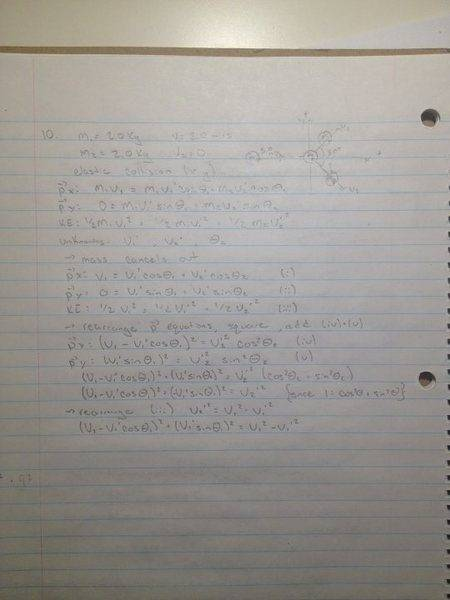# Momentum and Kinetic Energy, Elastic Collision

## Homework Statement

[/B]
A 2.0 kg ball moving with a speed of 3.0 m/s hits, elastically, an
identical stationary ball as shown. If the first ball moves away
with angle 30° to the original path, determine:
a. the speed of the first ball after the collision.
b. the speed and direction of the second ball after the
collision.

## Homework Equations

px: m1v1=m1v'1cosΘ1+m2v'2cosΘ2
py: 0=m1v'1sinΘ1+m2v'2sinΘ2
KE: 1/2m1v1^2=1/2m1v'1+1/2m2v'2

where I am using 1 and 2 to denote the first and second balls. and the "prime's" denoting final speed.
Θ1=30°
Since the balls have identical mass, I believe mass cancels out of the above equations.

## The Attempt at a Solution

[/B]
What I tried to do was to find the components of the vectors of final motion, use the conservation of momentum and kinetic energy to create three equations to solve for the three unknowns (Θ2, v'1, v'2).

I cancelled the mass out of all three equations, rearranged so that the Θ2 terms are on the same side of the momentum equations. I then squared the momentum equations and added them so that I could use the identity cos^2Θ+sin^2Θ=1 to get rid of the Θ2 terms and only have to solve for v'1 and v'2 using the KE equation and the added momentum equations. This is where I am getting stuck with the algebra:

(v1-v'1cos(30))^2+(-v'1sin(30))^2=(v'2)^2
and v'2=(v1)^2-(v'1)^2
so, subbing in for v'2:
(v1-v'1cos(30))^2+(-v'1sin(30))^2=(v1)^2-(v'1)^2

and at this point I should be able to solve for v'1, but i cant figure it outharuspex
Homework Helper
Gold Member
2020 Award
Just expand the terms and simplify (a lot).

•cassie123
Just expand the terms and simplify (a lot).

I gave that shot and ended up with v'1=2.598075 by using the quadratic equation.
This makes v'2=1.5 and Θ2=60°.

Does that make sense?

haruspex
Homework Helper
Gold Member
2020 Award
I gave that shot and ended up with v'1=2.598075 by using the quadratic equation.
This makes v'2=1.5 and Θ2=60°.

Does that make sense?
Looks right. You'll get more insight (and a heap of other benefits) if you resist the temptation to plug in numbers until the final step. Writing the original angle as theta, instead of 30 degrees, you find v1'=v1cos(theta), v2'=v1sin(theta).

ehild
Homework Helper
Yes.